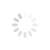# Increasing trend of students towards Mathematics through Mathematical fairs

Written By: / Monday, 27 June 2022 17:45

The word ‘fair’ in Punjabi culture has its own significance. The fair encourages a sense of happiness, excitement and something. The fair, especially for gentle babies taking knowledge of directly and indirectly, is very well aware of some important facts and makes the mind calm. School students have often heard of fair fairs, Baisakhi fairs, science fairs and have never seen it. But the students of mathematical festivals think differently in a different mood that the mathematical festivals will happen, actually reducing the undeclared scars of the student towards the mathematical fair. According to common belief, learning and teaching mathematics is considered difficult. Mathematical experts believe that connecting mathematics with daily life and using cleverness, understanding the basic concepts of mathematics and repeating them can yield good results for understanding mathematics. In order to teach mathematics, the teacher often uses mathematical calculations, grits, various figures, examples of daily living, to teach mathematical calculations in his class. By looking at, many brilliant students also find new ways to understand the facts of mathematics and enjoy explaining other students.
As a result students are understood by the group we know with peear learning.The Department of Education, the students’ mathematical fair is an attempt. In which the students will enjoy their resolved mathematical concepts by showing their own experiences with their own efforts in their own language, and directly or indirectly to their fellow students who need to understand the mathematical concepts Teach them Mathematical fair will be an exciting way of explaining analytical form to the students of the school, explaining the reasoning topic, the reasoning in a consistent way, and explaining how to combine mathematics in daily life. Let’s make a success of this effort by all the students, math students, maths & science teachers, learners, officers, fair attendants, and make this effort of the education department’s success and the mood of the student’s gentle mind and fear of mathematics Count it. In the fair, brain exercise puzzles, where concepts of mathematics are hidden, discussion of the works of great mathematicians will prove to be effective in increasing the trend towards students’ mathematics. To give practical information about students easily through mathematical subjects, The fair will prove effective.